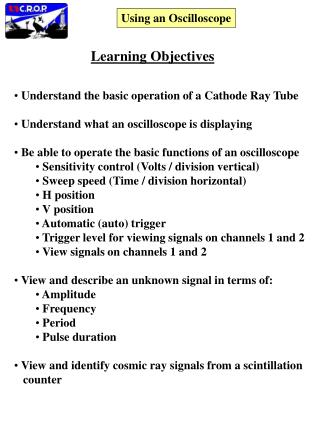# Using an Oscilloscope - PowerPoint PPT PresentationDownload PresentationUsing an Oscilloscope

Using an OscilloscopeDownload Presentation## Using an Oscilloscope

- - - - - - - - - - - - - - - - - - - - - - - - - - - E N D - - - - - - - - - - - - - - - - - - - - - - - - - - -
##### Presentation Transcript

1. Using an Oscilloscope Learning Objectives • Understand the basic operation of a Cathode Ray Tube • Understand what an oscilloscope is displaying • Be able to operate the basic functions of an oscilloscope • Sensitivity control (Volts / division vertical) • Sweep speed (Time / division horizontal) • H position • V position • Automatic (auto) trigger • Trigger level for viewing signals on channels 1 and 2 • View signals on channels 1 and 2 • View and describe an unknown signal in terms of: • Amplitude • Frequency • Period • Pulse duration • View and identify cosmic ray signals from a scintillation • counter

2. Using an Oscilloscope Outline • Introduction

3. Using an Oscilloscope Introduction • Oscilloscopes are common, useful tools for viewing electric • signals • An electric signal is the flow of charge through a conductor • An oscilloscope displays the voltage which is generated across • a resistor (50 ohms or 1 megaohm) as a function of time as the • electric signal passes through the resistor • How is the voltage related to the flow of charge in the • electric signal? • Current = charge flowing past a point (or through a resistor) • per unit time. • Units = charge / time = coulombs / second = Amperes • Variable used is I • Ohm’s law V = I  R ; V = voltage, R = resistance

4. Using an Oscilloscope H position Trigger level Display (Cathode Ray Tube) V position Voltage Time Sweep speed (Time / division, horizontal) Channel 1 Input Channel 2 Input Sensitivity controls (Volts / division, vertical)

5. Using an Oscilloscope Cathode Ray Tube

6. Using an Oscilloscope Slide template